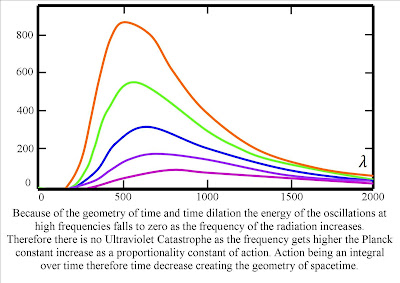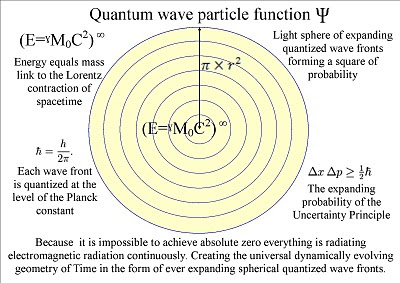Thursday, 18 March 2010

Euler Identity within a new quantum theory

The symmetry of light and variable of time within Quantum Atom Theory

~Time is a continuous process of the wave function collapsing and reforming. Forming new photons in space and new moments in time. That is part of the arrow of time or the time continuum that forms three dimensional spacetime.

~There is no time at the quantum level of the atoms and we have time independent quantum mechanics when the atom is not undergoing any chemical reaction. Therefore a quantum particle is still bran new after a half-life and all we have is a quantum wave particle function of possibilities.
~

~Heisenberg Uncertainty Principle and the Measure Problem are within an expanding light sphere. Therefore we see Pi in the equation for the reduced Planck constant and we have Einstein’s curvature of spacetime.

~Time moves at the speed of light and energy or mass slow it down to form their own spacetime geometry. Therefore the greater the energy of an object the shorter the time period relative to an observers own frame of reference. From the observers frame of reference time will be inverse like multiplication and division or frequency and wavelength. This is very difficult to visualize but in the same way that we have the Inverse Square Law in gravity because the atoms are at the centre of the gravitational field. We see time as inverse because the observer is at the centre of their own quantum field or reference frame (a sphere will look inverse from the centre looking out).
~We see time as linear because there was an evolutionary advantage in seeing time this way. But time is a measurement and a variable in three dimensional spacetime that forms the Lorentz contraction of spacetime. In Quantum Atom Theory at very small distances and short time scales this creates the Measure Problem of quantum physics.

~There is no ultraviolet catastrophe because time is only relative to the energy or mass of the object forming the spacetime. This is because the greater the energy the higher the frequency the shorter the wavelength and therefore the shorter the time period relative to an observers own created spacetime or reference frame.

~Quantum Atom theory is a visual theory and is sadly not based on mathematics. But it can take the most beautiful equations to come out of the mind of Man and link then into a coherent structure within geometry of space and time.
~In three dimensional space light will move in straight line in all directions forming light spheres. This will create a square of probability that the observer will see as the future uncertainty of everyday life and as Heisenberg Uncertainty Principle at the quantum level.
~The quantum wave particle function will expand as an inverse volume of space in the form of a sphere. This will place Euler Identity at the heart of creation of space and time. Therefore human mathematics and geometry is just a reflection of our physical Universe and the irrational number sequence that goes on forever and never repeats always forming something different represents the forward momentum of time.
~

In this theory consciousness does not collapse the wave function of quantum physics only physical action creating a photon electron coupling can do this. Consciousness is beyond the scope of this theory but consciousness must be governed by the same laws of physics as the rest of the Universe. But if time dilation can occur within the mind of the observer then the observer could be looking at a reflection of his or her own reality. This could one day explain why some people have dreams that come true or why meditation and praying seems to effect reality.
~

Link to Quantum Atom Theory on You Tube

~

Sunday, 14 March 2010

The relativity and probability of Time

Video The relativity and probability of Time.
~

Quantum Atom Theory is a theory on the dynamics of Light and Time that forms the geometry of spacetime. In this theory the individual atoms are creating their own Time by the emission and absorption of electromagnetic radiation (light). The atoms bound together forming their own spacetime geometry therefore the observer as a group of atoms is also creating their own spacetime geometry relative to their position and momentum.

Therefore the observer is the only true reference frame and we have relativity. The probability of the Uncertainty Principle is the same probability that the observer will have with any future event because time is the Hidden Variable. We know time is a variable because we have time dilation when objects accelerate towards the speed of light and also around objects of great mass.

Time is a measurement and a variable because we have Lorentz factor and the Lorentz contraction of space that changes the geometry of spacetime. Therefore we have the Measurement Problem because time is a measurement and a variable and the probability of quantum physics is the same probability that the observer will have with any future event.

In this theory we only need three dimensions and one variable of time. The parallel universes of Hugh Everett and the multiple dimension of String Theory are just individual space-times within our one three dimensional Universe. These space-times are on every level of creation from the Planck constant to objects of our everyday lives. Time is only relative to the energy or mass of the object forming the spacetime.

We have Quantum Entanglement because light has spherical symmetry and therefore photons will have opposite spin when they are on opposite sides of the light sphere. This also explains why pi is often combined with the Planck constant and is also in Heisenberg uncertainty equation.

Therefore the dynamics of light will form a universal process of symmetry forming and breaking for the whole Universe. This will create a mathematical base for the evolution of life. It is because life can choose when and where to collapse the wave function breaking the symmetry that we have freewill. Life will create its own ripples in the fabric of spacetime forming its own broken symmetry of its own evolutionary path.

In Quantum Atom Theory the outward momentum of light or EMR creates the inward force of gravity. When EMR comes in contact with another object it will be absorbed in proportion to the mass of that object. This will create an unbalanced force and the two objects will resonate together.

Because atoms consist mostly of empty space EMR of short wave lengths like x-rays can penetrate the objects. Therefore every single part of matter can take part in the gravitational interaction. The gravitation field will propagate at the same speed that EMR moves, the speed of light therefore there is no instantaneous action at a distance.

We have the inverse square law because the surface area of the light sphere increases with the square of the radius. Thus the strength of the gravitational field is inversely proportional to the square of the distance from the source.

This is my theory I am only an artist and therefore outside of the scientific community because of this there is no peer review. But any help promoting this theory on in the scientific community will be gratefully welcomed.

The aim of this theory is the unification of quantum and classical physics. Too see behind the paradoxes and probability to see the true simplicity and scientific beauty of our Universe. Those that look upon this theory and comprehend will see their own infinity without end.

~Euler Identity is at the heart of Quantum Atom Theory.

~

The reason the quantum wave particle function is inverse is because the observer is forming his own wave function observering the sphere from the inside looking out. Therefore the curvature of the sphere is expanding but also inverse.

~